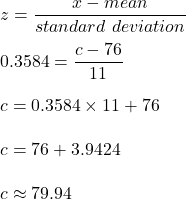## A class is given an exam. The distribution of the scores is normal. The mean score is 76 and the standard deviation is 11. Determine the tes

Question

A class is given an exam. The distribution of the scores is normal. The mean score is 76 and the standard deviation is 11. Determine the test score, c c , such that the probability of a student having a score greater than c c is 36 % 36% . P ( x > c )

in progress 0
4 months 2021-09-05T16:59:42+00:00 1 Answers 9 views 0

1. Answer: required value of c = 79.94.

Step-by-step explanation:

Let x denotes the exam score.

Given: The mean score is 76 and the standard deviation is 11.

to detrmine c , such that the probability of a student having a score greater than c  is 36 %.

or P(x>c)=0.36

Using z-score table , we get

z= 0.3584 [z-value corresponds to p-value of 0.36(one-tailed) is 0.3584]

Formula for z:hence, required value of c = 79.94.